# SUMIFS Function in Excel

Sums all data based on multiple criteria

## What is the SUMIFS Function in Excel?

We all know the SUMIF function allows us to sum the data given based on associated criteria within the same data. However, the SUMIFs Function in Excel allows applying multiple criteria.

### Formula used for the SUMIFS Function in Excel

“SUMIFS ( sum_range, criteria_range1, criteria1, [criteria_range2, criteria2, criteria_range3, criteria3, … criteria_range_n, criteria_n] )”

Where:

Criteria_range1 = Range of cells that we want to apply criteria1 against

Criteria1 = Used to determine which cells to add. Criteria1 is applied against criteria_range1.

Criteria_range2, criteria2, … =  The additional ranges along with their associated criteria.

The SUMIFS Function in Excel allows us to enter up to 127 range/criteria pairs for this formula. Remember:

• SUMIFS will return a numeric value.
• Rows and columns should be the same in the criteria_range argument and the sum_range argument.

Let’s take an example to understand it.  Suppose we use data about flowers and their cost per dozen for different regions. If I need to find out the total cost for Carnations for the South region, I can do it in the following manner: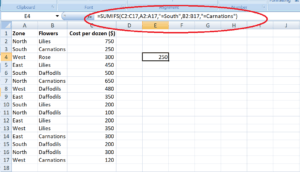Similarly, if I wish to find out the total cost of Daffodils for the North region, I can use the same formula.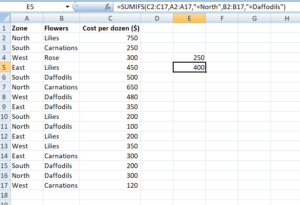Suppose I wish to find out the total cost of flowers for the East region, the formula to be used would be: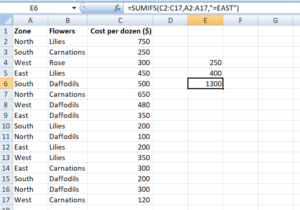The SUMIFS function can use comparison operators like ‘=’, ‘>’, ‘<‘.  If we wish to use these operators, we can apply them to an actual sum range or any of the criteria ranges. Also, we can create comparison operators using them:

• ‘<=’ (less than or equal to)
• ‘>=’ (greater than or equal to)
• ‘<>’ (less than or greater than/not equal to)

Let’s take an example to understand this in detail.

Using the sales figures per region of different salespersons, I wish to find out the:

1. Sales of North region greater than 100
2. Sales greater than 500

I can use the abovementioned operators as follows:

##### Sales of North region greater than 100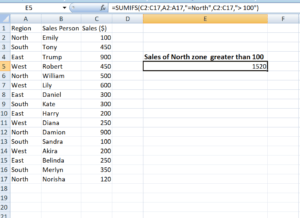##### Sales greater than 500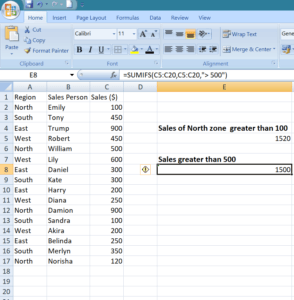### Use of wildcards

Wildcard characters such as  ‘*’ and ‘?’ can be used within the criteria argument when using the SUMIFS function. Using these wildcards will help us in finding matches that are a similar but not accurate match.

Asterisk (*) – It matches any sequence of characters. It can be used after, before or surrounding criteria to allow partial search criteria to be used.

For example, if I apply the following criteria in SUMIFS function:

• N* – It implies all cells in the range that start with N
• *N – It implies all cells in the range that ends with N
• *N* – Cells that contain N

Question mark (?) – It matches any single character. Suppose I apply N?r as the criteria. Here “?” will take the place of a single character. N?r will match with North, Nor, etc. However, it will not take into consideration Name.

What if the given data contain an asterisk or an actual question mark?

In this case, we can use “tilde (~)”. We need to type “~” in front of the question mark in that scenario.

### Using named ranges with SUMIFS Function

Named range is the descriptive name of a collection of cells or range in a worksheet. We can use named ranges while using the SUMIFS function.

### SUMIF vs. SUMIFS

• When using SUMIF, we can evaluate only one condition, whereas different criteria can be evaluated under SUMIFS formula. This is the primary difference between the two Excel functions.
• SUMIFS is available from MS Excel 2007.
• The SUMIF function can be only used for adding a single continuous range based on a single specified range with a single criterion, whereas, SUMIFS can be applied over multiple continuous ranges.

### Free Excel Course

Don’t forget to check out CFI’s Free Excel Crash Course to learn more about functions and develop your overall Excel skills. Become a successful financial analyst by learning how to create sophisticated financial analysis and financial modeling.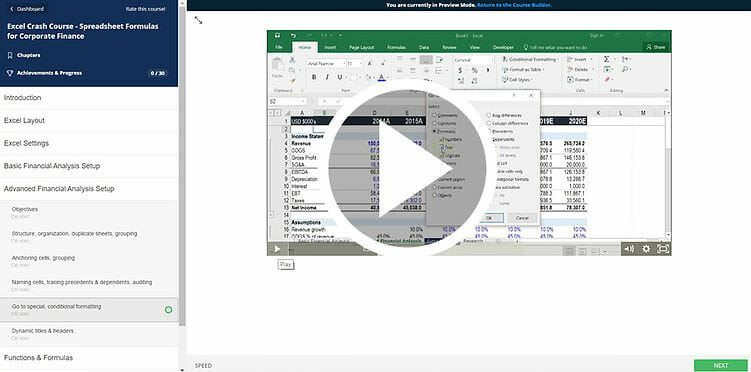Thanks for reading CFI’s guide to important Excel functions! By taking the time to learn and master these functions, you’ll significantly speed up your financial analysis. To learn more, check out these additional CFI resources:

• Excel Functions for Finance